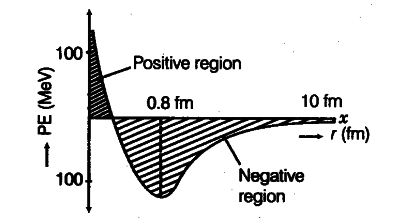# Draw a plot of potential energy between a pair of nucleons

Draw a plot of potential energy between a pair of nucleons as a function of their separation. Mark the regions where potential energy is (i) positive and (ii) negative.

Plot the graph between the potential energy of a pair of nucleons as a function of their separation.(i) For distance less than 0.8 fm, negative PE decreases to zero and then becomes positive.
(ii) For distances larger than 0.8 fin, negative PE goes on decreasing.